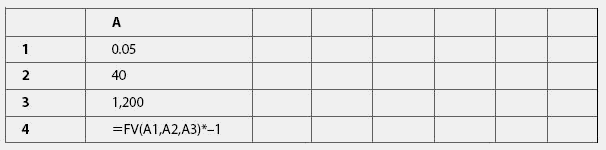# compound interest

(redirected from Annual Equivalent Rate (or AER) as used in the UK)
Also found in: Dictionary, Thesaurus, Legal, Encyclopedia.

## Compound interest

Interest paid on previously earned interest as well as on the principal.

## Compound Interest

The interest on a loan or other fixed-income instrument where interest previously paid is included in the calculation of future interest. For example, if one has a certificate of deposit for \$1,000 that pays 3% in interest each month, The interest paid in the first month is \$30 (3% of \$1,000), in the second month it is \$30.90 (3% of \$1,030), and so forth. The more frequently interest is compounded, the higher the yield will be on the instrument. See also: Amortization.

## compound interest

Interest paid both on principal and on interest earned during previous compounding periods. Essentially, compounding involves adding interest to the sum of principal and any previous interest in order to calculate interest in the next period. Compare simple interest. See also frequency of compounding.

## Compound interest.

When the interest you earn on an investment is added to form the new base on which future interest accumulates, it is compound interest.

For example, say you earn 5% compound interest on \$100 every year for five years. You'll have \$105 after one year, \$110.25 after two years, \$115.76 after three years, and \$127.63 after five years.

Without compounding, you earn simple interest, and your investment doesn't grow as quickly. For example, if you earned 5% simple interest on \$100 for five years, you would have \$125. A larger base or a higher rate provide even more pronounced differences.

Compound interest earnings are reported as annual percentage yield (APY), though the compounding can occur annually, monthly, or daily.

## compound interest

see INTEREST.
Collins Dictionary of Business, 3rd ed. © 2002, 2005 C Pass, B Lowes, A Pendleton, L Chadwick, D O’Reilly and M Afferson

## compound interest

the INTEREST on a LOAN that is based not only on the original amount of the loan but the amount of the loan plus previous accumulated interest. This means that, over time, interest charges grow exponentially; for example, a £100 loan earning compound interest at 10% per annum would accumulate to £110 at the end of the first year and £121 at the end of the second year, etc., based on the formula:

compound sum = principal (1 + interest rate") number of periods that is, 121 = 100 (1 + 0.1)2. Compare SIMPLE INTEREST.

Collins Dictionary of Economics, 4th ed. © C. Pass, B. Lowes, L. Davies 2005

## compound interestThe process of charging, or earning, interest on interest. Interest accrued in prior periods is added to the principal,and then interest in the current period is calculated on the total.

Example: If you saved \$100 per month, starting at age 25, at an earnings rate of 5 percent per annum, you would have \$144,959 by age 65, only \$48,000 of which would be money you contributed. To perform your own calculations, use the following formula to create a Microsoft Excel spreadsheet:

FV(Interest Rate, Number of Years, Savings per Year)*-1

For Interest Rate enter the cell address where you will note the interest rate you expect to earn; for Number of Years enter the cell address where you enter the number of years you expect to save the same amount each year; and for Savings per Year enter the cell address where you will enter the amount you expect to save each year.

The Complete Real Estate Encyclopedia by Denise L. Evans, JD & O. William Evans, JD. Copyright © 2007 by The McGraw-Hill Companies, Inc.
Site: Follow: Share:
Open / Close